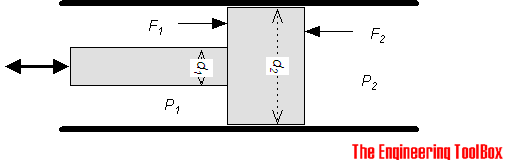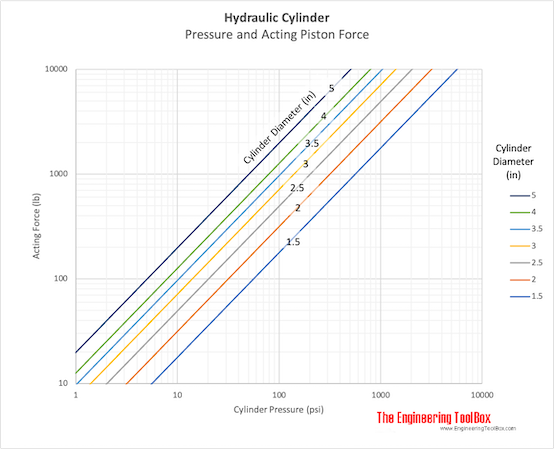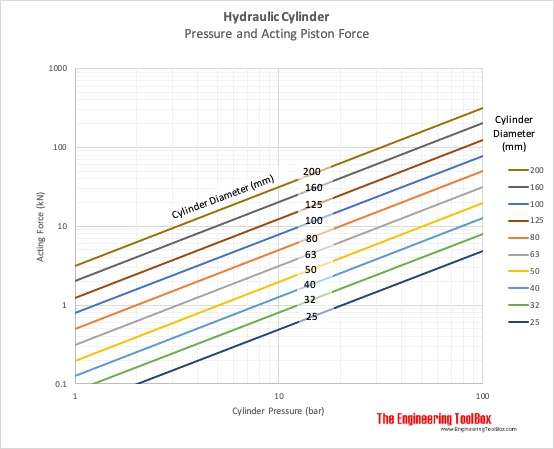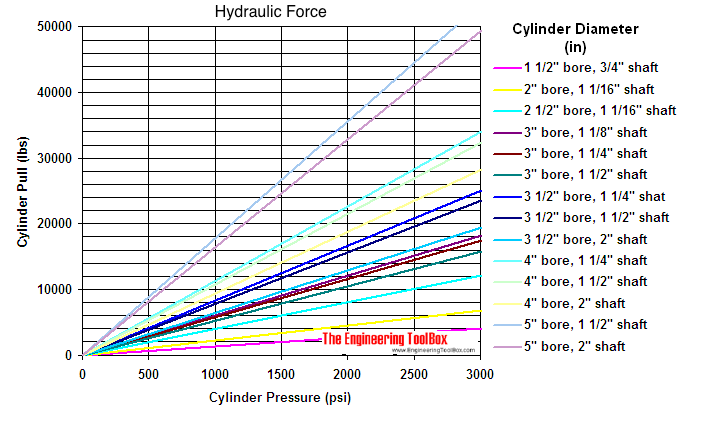Engineering ToolBox - Resources, Tools and Basic Information for Engineering and Design of Technical Applications!

# Hydraulic Force vs. Pressure and Cylinder Size

## Calculate hydraulic cylinder force.The force produced on the rod side (1) of a double acting hydraulic piston - can be expressed as

F1 = P1 (d22 - d12) / 4)                                    (1)

where

F1 = rod force (lb, N)

d1 = rod diameter (in, mm)

d2 = piston diameter (in, mm)

P1 = pressure in the cylinder on the rod side (psi, N/mm2)

• 1 bar = 105 N/m2 = 0.1 Nm/mm2

The force produced on the opposite of rod side (2) - can be expressed as

F2 = P2 (π d22 / 4)                                   (2)

where

F2 = rod force (lb, N)

P2 = pressure in the cylinder (opposite rod) (psi, N/mm2)

### Hydraulic Force Calculator

#### Imperial Units

##### Pressure acting on rod side

Piston diameter - d2 (in)

Rod diameter - d1 (in)

Cylinder pressure - P1 (psi)

##### Pressure acting on opposite of rod side

Piston diameter - d2 (in)

Cylinder pressure - d2 (psi)

#### Metric Units

##### Pressure acting on rod side

Piston diameter - d2 (mm)

Rod diameter - d1 (mm)

Cylinder pressure - P1 (bar)

##### Pressure acting on opposite of rod side

Piston diameter - d2 (mm)

Cylinder pressure - d2 (bar)

### Related Mobile Apps from The Engineering ToolBox

- free apps for offline use on mobile devices.

### Rod Force F2 diagram

- when pressure act on opposite side of rod.

#### Imperial Units###• 1 psi (lb/in2) = 144 psf (lbf/ft2) = 6,894.8 Pa (N/m2) = 6.895x10-3 N/mm2 = 6.895x10-2 bar
• 1 N/m2 = 1 Pa = 1.4504x10-4 lb/in2 = 1x10-5 bar = 4.03x10-3 in water = 0.336x10-3 ft water = 0.1024 mm water = 0.295x10-3 in mercury = 7.55x10-3 mm mercury = 0.1024 kg/m2 = 0.993x10-5 atm
• 1 lbf (Pound force) = 4.44822 N = 0.4536 kp
• 1 N (Newton) = 0.1020 kp = 7.233 pdl = 7.233/32.174 lbf = 0.2248 lbf = 1 (kg m)/s2 = 105 dyne = 1/9.80665 kgf
• 1 in (inch) = 25.4 mm
• 1 m (meter) = 39.37 in = 100 cm = 1000 mm

### Rod Force F1 diagram

- when pressure act on the same side of the rod.

#### Imperial Units## Related Topics

• ### Hydraulics and Pneumatics

Hydraulic and pneumatic systems - fluids, forces, pumps and pistons.

## Related Documents

• ### BFD - Block Flow Diagram

A Block Flow Diagram is a schematic illustration of a major process.
• ### Force Ratio

The force ratio is the load force versus the effort force.
• ### Hydraulic Cylinder Area vs. Diameter

Typical hydraulic cylinders and acting area vs. cylinder diameter.
• ### Hydraulic Cylinders - Volume Displacement

The volumetric displacement of hydraulic cylinders.
• ### Hydraulic Force vs. Pascal's Law

Pascal's law and the hydraulic force acting in fluids.
• ### Hydraulic Oil Pump - Horsepower vs. Pressure and Volume Flow

The power required for hydraulic pumping.
• ### Hydraulic Pump Motor Sizing

Motor size vs. flow rate, shaft torque, shaft power and hydraulic power.
• ### Hydraulic Pump Volume Capacity

Calculate hydraulic pump volume capacity.
• ### Hydrostatic Pressure vs. Depth

Depth and hydrostatic pressure.

## Engineering ToolBox - SketchUp Extension - Online 3D modeling!

Add standard and customized parametric components - like flange beams, lumbers, piping, stairs and more - to your Sketchup model with the Engineering ToolBox - SketchUp Extension - enabled for use with older versions of the amazing SketchUp Make and the newer "up to date" SketchUp Pro . Add the Engineering ToolBox extension to your SketchUp Make/Pro from the Extension Warehouse !

We don't collect information from our users. More about

## Citation

• The Engineering ToolBox (2009). Hydraulic Force vs. Pressure and Cylinder Size. [online] Available at: https://www.engineeringtoolbox.com/hydraulic-force-calculator-d_1369.html [Accessed Day Month Year].

Modify the access date according your visit.

9.19.12